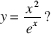# AP Calculus BC Practice Test 18

### Test Information9 questions18 minutes

Calculator Disallowed

1. The graph below consists of a quarter-circle and two line segments, and represents the velocity of an object during a 6-second interval.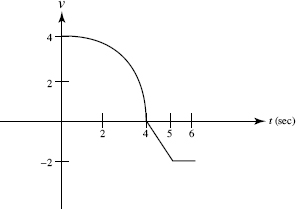The object's average speed (in units/sec) during the 6-second interval is

2. The graph below consists of a quarter-circle and two line segments, and represents the velocity of an object during a 6-second interval.The object's acceleration (in units/sec2) at t = 4.5 is

3.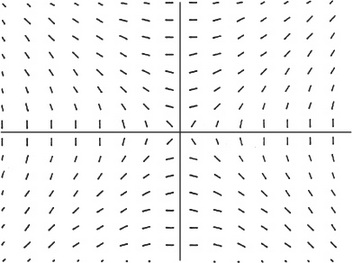Which of the following equations can be a solution of the differential equation whoseslope field is shown above?

4. If y is a differentiable function of x, then the slope of the curve of xy2 - 2y + 4y3 = 6 at the point where y = 1 is

5. For the function f shown in the graph, which has the smallest value on the interval 2 ≤ x ≤ 6?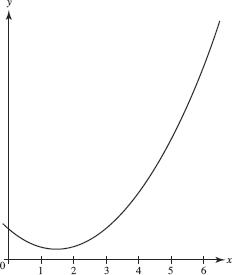6. The table shows some values of a differentiable function f and its derivative f :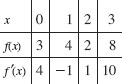7. The solution of the differential equation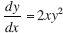for which y = -1 when x = 1 is

8. The base of a solid is the region bounded by the parabola y2 = 4x and the line x = 2. Each plane section perpendicular to the x-axis is a square. The volume of the solid is

9. Which of the following could be the graph of